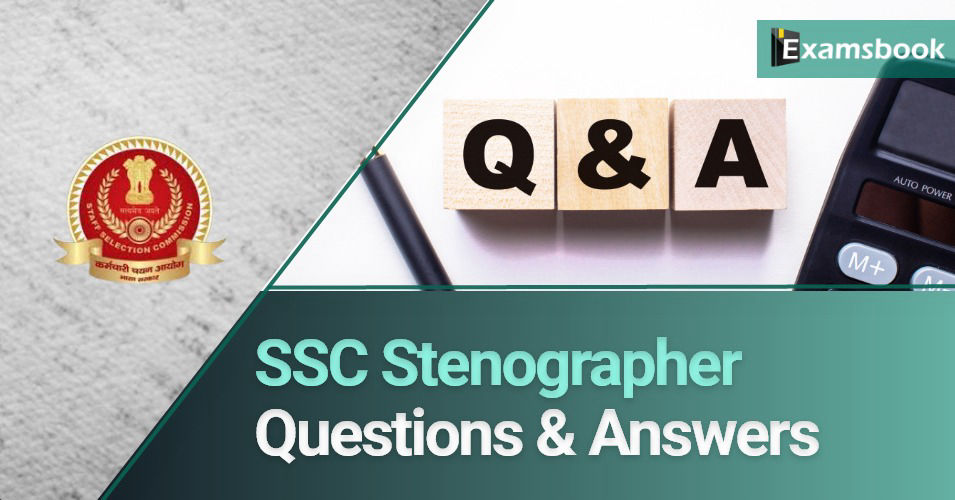• Save

# SSC Stenographer Question and AnswerThe need of SSC Stenographer remains in every government department. Due to this, it is becoming a very popular job. If you are preparing for SSC Steno then you must practice the questions given here. These questions have been designed keeping in mind the exam syllabus. Practicing these questions will increase your score. These given questions are the questions which have come in the previous examinations. So you must practice these questions

## Important SSC Stenographer Questions

Q :  Ram can complete ¼ th part of work in 8 days and Shyam can complete 2/4 th of the work in 6 days and Naresh can complete ¾th the work in 12 days. In how many days can they together complete it?

(A) $$6 {11 \over 17 }$$

(B) $$5 {11 \over 17 }$$

(C) $$8 {11 \over 17 }$$

(D) $$7 {11 \over 17 }$$

Q :  A, B and C can complete a work in 10, 15 and 20 days respectively. A and B start it together. B is replaced by C after 2 days. A leave it after one more day. In how many days work was completed?

(A) 13

(B) $$13 {1 \over 13 }$$

(C) $$18 {4 \over 3 }$$

(D) 18

Q :  A complete a work at four times the speed than C. If together they can complete a work in 20 days, in how many days can A alone complete it?

(A) 25

(B) 100

(C) 75

(D) 80

Q :  30% of first number is 75% of the second number. What is the respective ratio of the first number to the second number?

(A) 5:2

(B) 5:3

(C) 25:14

(D) 15:16

Q :  A cycle is sold for Rs.2070 at profit of 15%, at what profit of 15%, at what price it should be sold so as to earn 25% Profit.

(A) 2150

(B) 2250

(C) 2350

(D) 2450

Q :  If one object is sold for 5% instead of 5 % loss the seller gets Rs. 33.6 more. What is the cost Price?

(A) 336

(B) 454

(C) 672

(D) 785

Q :  Rekha is 25 years younger to her mother. Ten years back their ages were in the ratio of 1:6. Present age of mother is

(A) 25

(B) 40

(C) 35

(D) 15

Q :  Ram’s present age is one-sixth of his father’s age. If the difference between their present ages is 35 years, what is his father’s present age?

(A) 32 Years

(B) 42 Years

(C) 52 Years

(D) 44 Years

Q :  Present age ratio of A and B is 3:4 four years after it will be 11:14. What is B’s Present age?

(A) 24

(B) 18

(C) 22

(D) 28

Q :  The total number of students in a school is 3250. If the number of girls in the school is 1495, then what is the respective ratio of the total number of boys to the total number of girls in the school?

(A) 23:27

(B) 25:29

(C) 27:23

(D) 29:25